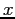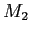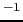Next: Running the test Up: Internal waves Previous: Initial conditions   Contents

### Boundary conditions

Boundary conditions are gradient-free on the temperature and salinity fields at the western boundary. This is implemented in the function `BoundaryScalars` in the file `boundaries.c` as

```for(k=grid->ctop[ib];k<grid->Nk[ib];k++) {
phys->boundary_T[jptr-grid->edgedist][k]=phys->T[ib][k];
phys->boundary_s[jptr-grid->edgedist][k]=phys->s[ib][k];
}
```
where `ib` is the index of the Voronoi point adjacent to the boundary edge `j`.

For the velocity field, the-direction velocity is specified at the western boundary as a sinusoidally varying barotropic velocity with atidal period of 12.42 hours, and the other components are zero. This is implemented in the function `BoundaryVelocities` in the file `boundaries.c` as

```for(k=grid->etop[j];k<grid->Nke[j];k++) {
phys->boundary_u[jptr-grid->edgedist][k]=
prop->amp*sin(prop->omega*prop->rtime);
phys->boundary_v[jptr-grid->edgedist][k]=0;
phys->boundary_w[jptr-grid->edgedist][k]=0;
}
```
The variables `amp` and `omega` are set in `suntans.dat` (`amp = 4` mm sand `omega = 1.4026e-4` rad s), and `rtime` is the simulation time, in seconds. These velocities are then used in the `OpenBoundaryFluxes` function to compute the velocity normal to the boundary face with
```for(k=grid->etop[j];k<grid->Nke[j];k++)
ub[j][k]=phys->boundary_u[jptr-grid->edgedist][k]*grid->n1[j]+
phys->boundary_v[jptr-grid->edgedist][k]*grid->n2[j];
```
Since there is only one boundary, no if-statements are required to determine if this is an open or closed boundary condition, such as in Section 6.5. More complex partially-clamped boundary conditions can be implemented that allow the internal wave field to exit the domain while still forcing the barotropic velocity field, but this is beyond the scope of this simplified example.Next: Running the test Up: Internal waves Previous: Initial conditions   Contents
2014-08-06